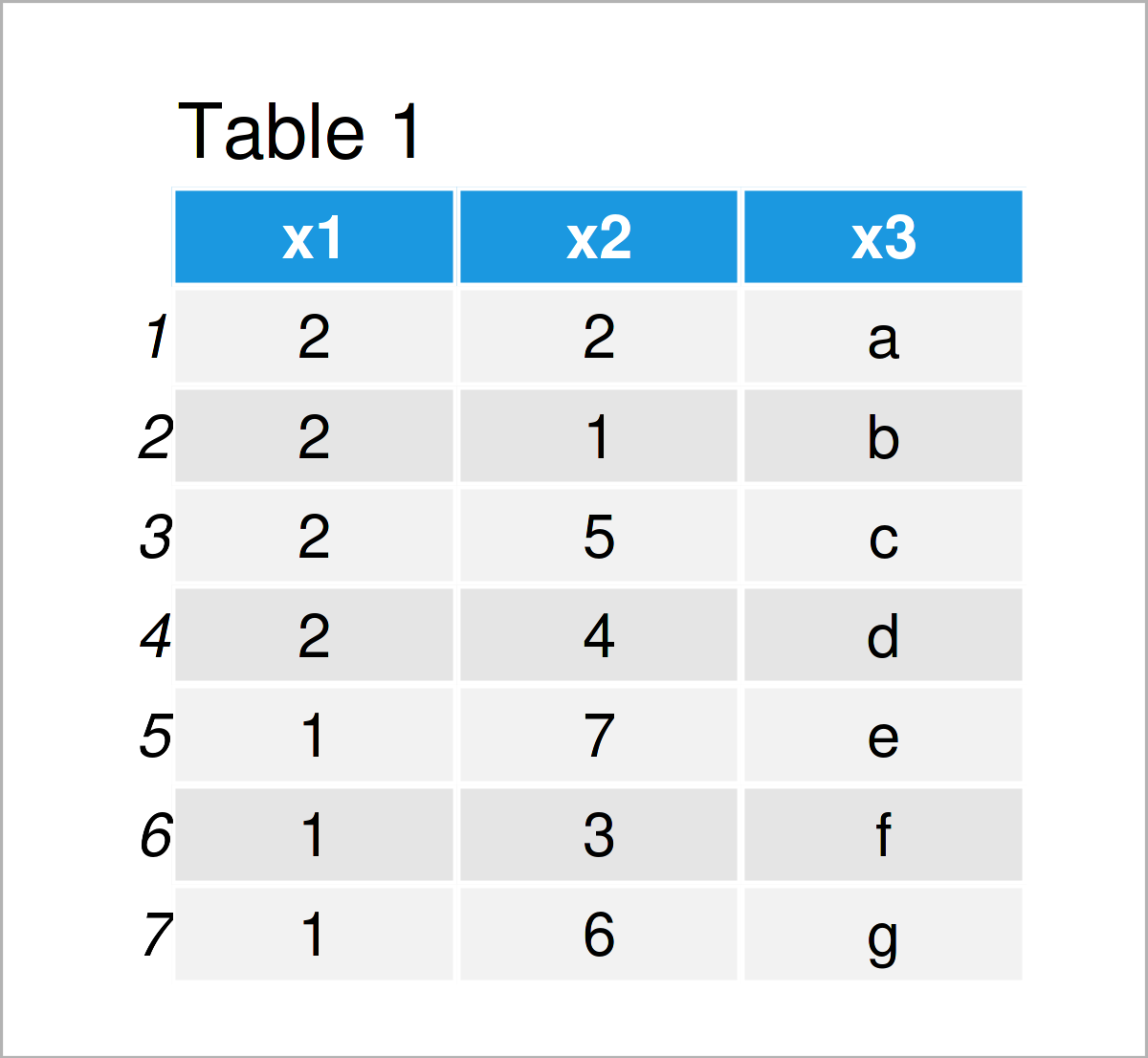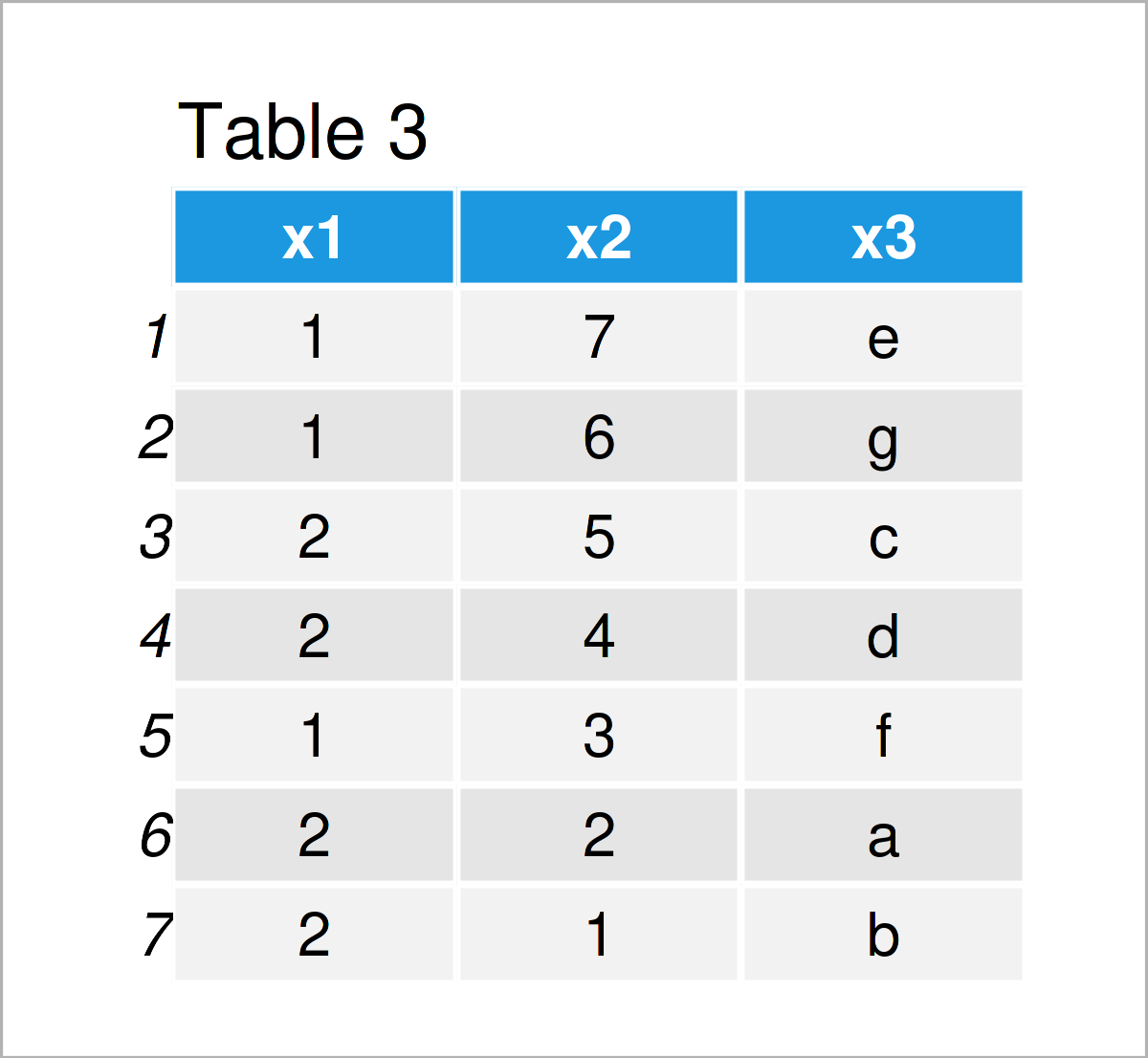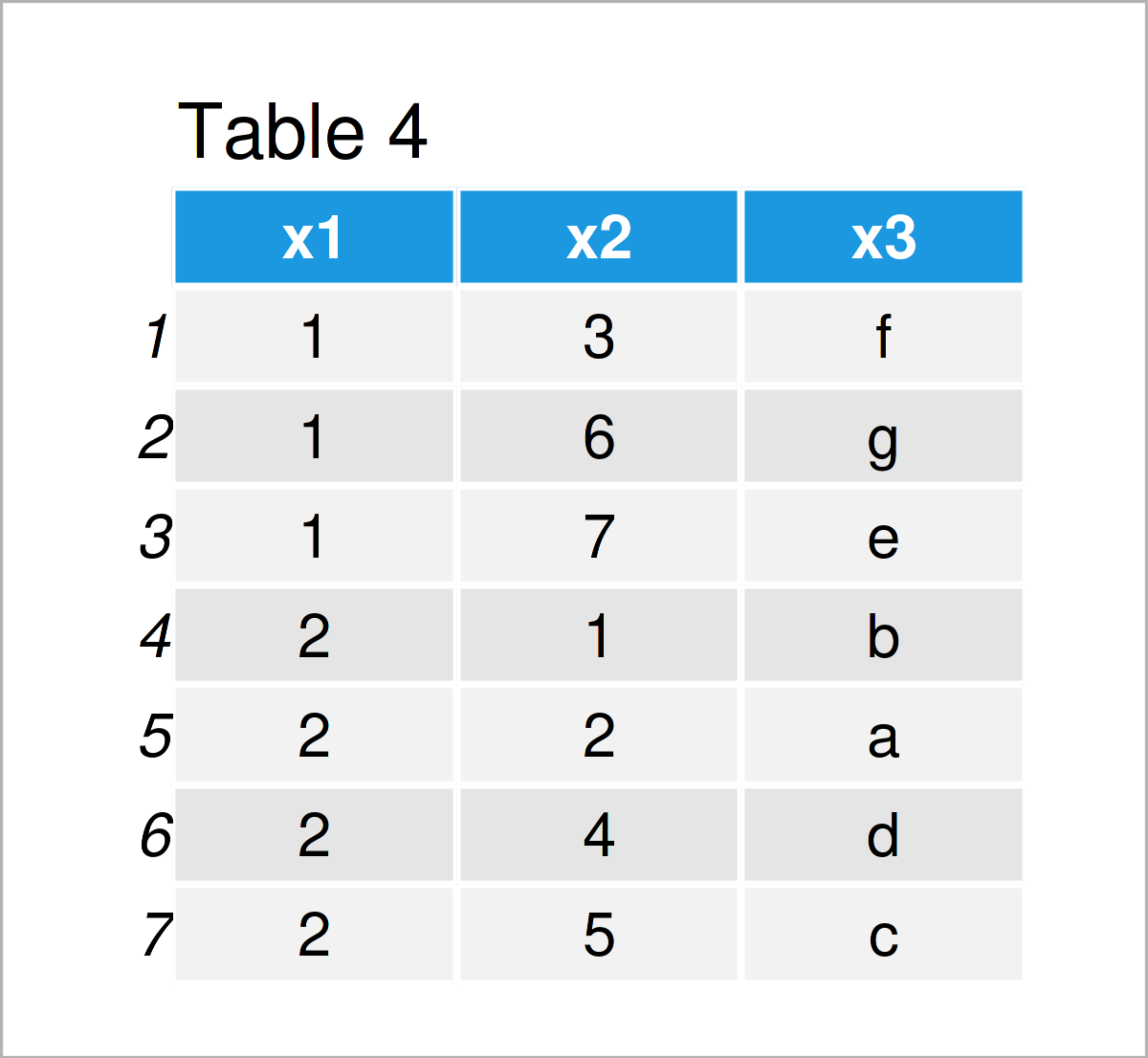# Sort Rows of data.table in R (3 Examples)

This tutorial demonstrates how to reorder the rows of a data.table in the R programming language.

So let’s dive into it!

## Example Data & Software Packages

First, we have to install and load the data.table package:

```install.packages("data.table")                     # Install data.table package

We’ll also have to create some example data:

```data <- data.table(x1 = c(2, 2, 2, 2, 1, 1, 1),    # Create data.table
x2 = c(2, 1, 5, 4, 7, 3, 6),
x3 = letters[1:7])
data                                               # Print data.table```Table 1 shows the structure of the example data.table: It contains seven rows and three columns.

## Example 1: Sort Rows of data.table Based on One Column

The following syntax shows how to order the rows of a data.table based on a single column of this data.table.

For this task, we can use the setorder function as shown below:

```data_new1 <- data                                  # Duplicate data.table
setorder(data_new1, cols = "x1")                   # Reorder data.table
data_new1                                          # Print updated data.table```The output of the previous R code is shown in Table 2: A new data.table where the rows have been sorted in ascending order according to the values in the variable x1.

## Example 2: Sort Rows of data.table in Descending Order

The first example has explained how to sort the rows of a data.table in ascending order.

This example, in contrast, explains how to order a data.table descendingly.

To achieve this, we have to specify a – sign in front of the variable based on which we want to sort.

In this specific example, we are ordering the rows of our data set based on the column x2:

```data_new2 <- data                                  # Duplicate data.table
setorder(data_new2, cols = - "x2")                 # Reorder data.table
data_new2                                          # Print updated data.table```By executing the previous R programming code, we have created Table 3, i.e. another data.table sorted in descending order based on the column x2.

## Example 3: Sort Rows of data.table Based on Multiple Columns

The following R programming code illustrates how to order the rows of a data.table based on multiple columns.

More precisely, the following R syntax orders our data.table using the two columns x1 and x2:

```data_new3 <- data                                  # Duplicate data.table
setorder(data_new3, cols = "x1", "x2")             # Reorder data.table
data_new3                                          # Print updated data.table```As shown in Table 4, we have created another version of our input data.table, this time sorted according to the column x1 and to the subgroups of x2.

## Video, Further Resources & Summary

I have recently released a video on the Statistics Globe YouTube channel, which shows the R programming code of this tutorial. You can find the video below.

Please accept YouTube cookies to play this video. By accepting you will be accessing content from YouTube, a service provided by an external third party.If you accept this notice, your choice will be saved and the page will refresh.

In addition, you may want to read the other R tutorials on my website:

Summary: You have learned in this tutorial how to sort the rows of a data.table in R. If you have additional questions, please tell me about it in the comments below.

Subscribe to the Statistics Globe Newsletter